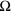## LEDs

First of all we need some electrical engineering fundamentals (based on https://electronicsclub.info/leds.htm):

I own several red and green LEDs, so I will use them for my demo. Every LED must have a resistor connected in series to limit the current flowing through the LED, to prevent it froming burning out instantly. The Resistor value can be calculated with a simple formular

 R = (VS – VL) / I

R = resistor value in ohms ().
VS = supply voltage.
VL = LED voltage (2V generally, or 4V for blue and white LEDs).
I = LED current in amps (A)

In our case, we will connect the LEDs to the RaspberryPi GPIOs which are operating at 3.3V (VS = 3.3V). We want our diode to be exposed to not more than 20mA (I = 0.02A). We are using red and green LEDs (VL=2.0V).

The Raspberry Pi can only source up to 5mA of current, meaning we must choose our resistor to limit the current to less than 5mA.

These figure let us calculate the resistors value to be: (3.3V-2.0V)/0.005A =  260 Ohm.

270Ohm is a standard resistor value, so lets go with it – you want to choose the closest standard resistor value that is greater than the theoretical value calculated before, to make sure the GPIO draw is less than 5mA.

## The board

I built my setup on a regular bread such as the one you find here:Be aware that the red and blue lines are connected along the board from column 1-<end>, while the non-colored holes are connected vertically from a-e and f-j. So you will need to bridge from one field to the other, as well as from the ground line to the building field. To brigde from one field into the other, I used a regular bridge on the way towards ground and a resistor on the way towards “+” .See the board in action with a 3.7 V Lipo pack I had in spare from one of my other projects.

Happy building!

Next step will be to connect the board to our Raspberry Pi. Stay tuned 😉